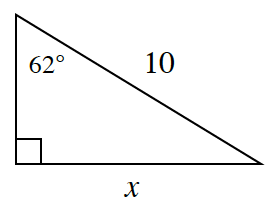### Home > GC > Chapter 9 > Lesson 9.2.4 > Problem9-94

9-94.

Multiple Choice: Solve this problem without a calculator: examine the triangle below. Find the approximate value of $x$. Use the values in the trigonometric table below as needed.$θ$ $\cosθ$ $\sinθ$ $\tanθ$ $28°$ $0.883$ $0.469$ $0.532$ $62°$ $0.469$ $0.883$ $1.881$
1. $4.69$

1. $5.32$

1. $8.83$

1. $18.81$

1. None of these

(c) because $\sin\left(62^{\circ}\right)=\frac{x}{10}$, so $0.883=\frac{x}{10}$. Isolate $x$, so $x\approx8.83$.